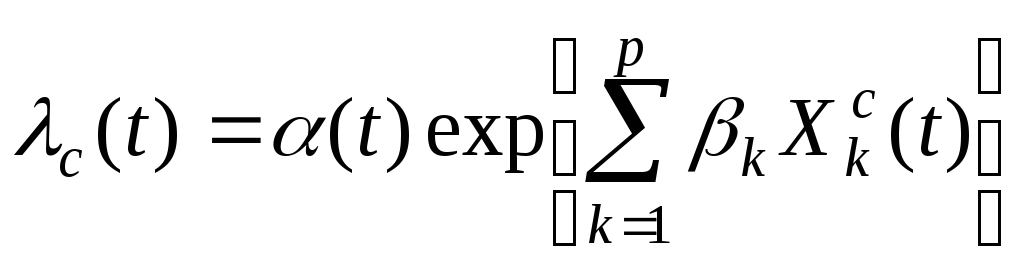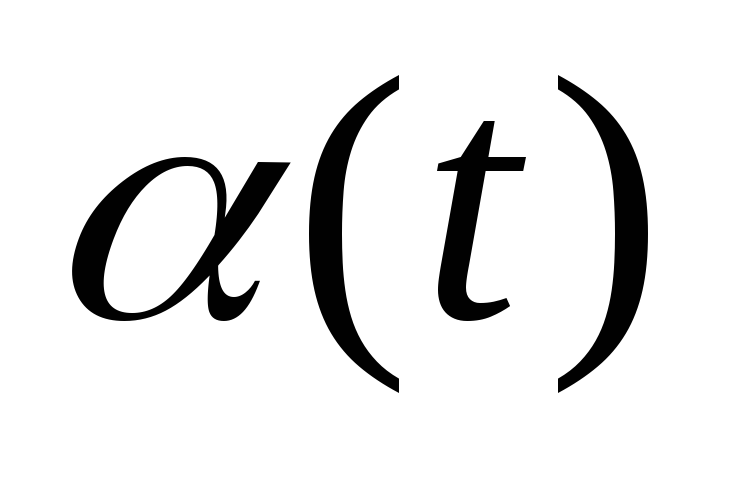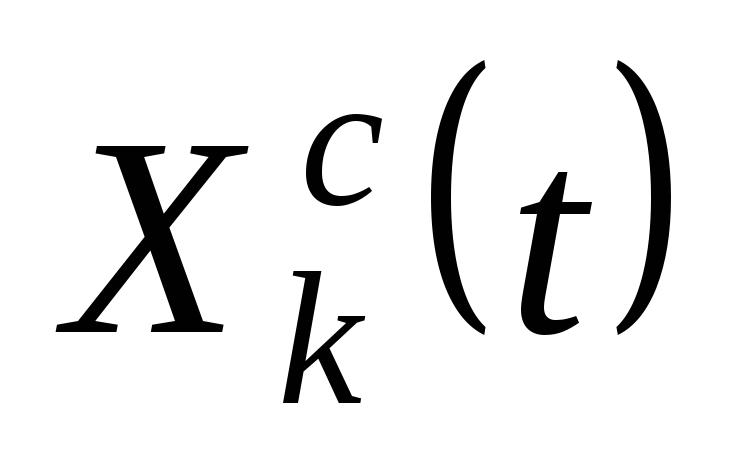# Empirically assessing the causes of civil war

Baixar 306,08 Kb.
 Página 4/5 Encontro 26.05.2017 Tamanho 306,08 Kb.
1   2   3   4   5

## Estimation techniques

Pooled cross-sectional models are a common form of econometric analysis of civil conflict. These models tend to rely on maximum likelihood estimators, probit, logit, etc. with corrections for temporal dependence.24 The fundamental problem regarding pooled time series analysis is that it assumes away unmeasured heterogeneity. Indeed such models rely on a strong assumption of homogeneity, which is untenable.

Fixed effects models have also been applied to the study of civil war. Such models are designed to account for fixed unobserved differences that stem from unmeasured heterogeneity, by assuming that differences across units can be captured in differences in the constant term. Thus in equation (1), each i is treated as an unknown parameter to be estimated. With the least squares dummy variable (LSDV) model this is typically done by creating a dummy variable for each country, thereby accounting for the unmeasured heterogeneity across units. The problem with fixed effects models for analyzing civil conflict (especially as a binary variable) is that so many units show no temporal variation on the dependent variables, hence having no impact on the parameter estimates. The use of fixed effect model to attempt to solve the problem of unmeasured heterogeneity results in a worse problem due to the rareness of the event. Beck & Katz condemn the use of fixed effects models with panels exhibiting the properties of civil conflict data, stating:

It is absurd to exclude over 90 percent of the cases from the analysis (or, equivalently, to allow them in the analysis but not allow them to affect the statistical results) and then conclude that some independent variable like democracy has the opposite effect of what every sensible study has shown…To include fixed effects in these analyses is never a good idea (Beck & Katz, 2001: 490).25
Collier & Hoeffler (2001) compare several different estimations, including a random effects model, a pooled logit with temporal dummies, rare events logit, and a fixed effects estimation. The results or their analysis are robust across estimations, except for the fixed effects model. Indeed, Collier & Hoeffler (2001) lost a number of cases in their fixed effects test due to the lack of variation in the dependent variable over time. The Uppsala data suffers less from the rare events problem in this regard, since ‘only’ 83% of the countries are thrown out of the analysis, but even at this level it seems that the fixed effects model as applied to a binary dependent variable is problematic.

Random effects models as applied to conflict assume that individual (country) specific constant terms are randomly distributed across the cross-sectional units. Such an assumption works best if the panel data is a sample of cross-sectional units drawn from a larger population. This is not the case for conflict data. In this regard, this may be a rather heroic assumption for conflict analysis.

Unlike the fixed effects approach, random effects treatments may still suffer from inconsistency due to omitted variables. The random effects approach treats the individual (country specific) effects as uncorrelated with other regressors.26 The Hausman test can be used to determine whether the individual effects are correlated with the regressors. With a low Hausman test value, the random effects approach is consistent and efficient. With a high Hausman test value, we are in trouble given the problematic nature of fixed effect estimators.

To address the problem of rare events, King & Zeng (2001) have devised a rare events logit estimator. Collier & Hoeffler (2001) find that this estimator produces almost the same results as the pooled logit with time dummies and the random effects test. By decreasing the ‘rareness’ of the event (i.e. lowering the threshold of what constitutes conflict), the need to correct for rareness is reduced, actually it probably made completely unnecessary.

To address a problem of endogeneity in their panel study Ebdawali & Sambanis (2002) extend the Rivers & Vuong (1988) framework for dealing with the potential endogeneity of continuous right-hand-side variables in probit models. This means estimating a two-stage probit model, which accounts for the lagged time structure and the endogeneity.

Hazard models have been applied to understanding the onset of civil war (Hegre et al., 2001) and the duration of civil war (Collier, Hoeffler & Söderbom, 2001) and Fearon (2001). Hegre et al., (2001) utilize a calendar-time proportional hazard, or Cox regression model (Cox, 1972) (Raknerud & Hegre, 1997). Since the Cox regression model is semi-parametric, it is not necessary to make assumptions about the shape of the baseline hazard function. One only needs to assume that the two hazard functions are proportional over time.27 Raknerud & Hegre (1997) adapt the Cox regression model to account for calendar time, in contrast to the typical application of proportional hazard models, which is to look at time at risk. The Cox regression method assumes that the hazard of an event (i.e. the onset of civil conflict) c(t) for country c can be factored into a parametric function of time-dependent risk factors and a non-parametric function of time itself, the baseline hazard:,(2)

whereis the baseline hazard.is an explanatory variable for country c, which could be time-dependent. k is the regression co­ef­ficient. Note that t is expressed in terms of calendar time – the number of days since the last exchange rate regime shift. This differs from most survival models, in which t is an expression of time at risk. This model imposes an exponential-regression distribution (exp(-t/alfa)), on the time elapsed, with a constant hazard rate. Hegre et al. (2001) do not explicitly account for unmeasured heterogeneity, observations are assumed to be independent and the variability associated with each observation is determined by a single dispersion factor, 2 or .28

Cox regression, proportional hazard models can be adapted to account for unmeasured heterogeneity (Beck & Katz, 2001). One technique is a split population model, in which some countries never are in civil war and others are at risk.29 Random effects and Bayesian hierarchical models are other alternatives.

Hazard models are well suited for duration analysis. Fearon (2001) determined that the differences between the results from using a Cox proportional hazard and the Weibull duration model were negligible. He does not do this, but one can account for unmeasured heterogeneity by using a Weibull duration model with gamma heterogeneity (Beck & Katz, 2001). Collier, Hoeffler & Söderbom (2001) account for duration dependence with a piecewise exponential estimation of the baseline hazard and control for unobserved country-specific heterogeneity with a random effect i (Heckman & Singer, 1984).

Civil conflict panel data is characterized by a set of data problems. Recent innovations in econometric estimation techniques allow conflict researchers to address these data problems and account for them in their analyses.

1   2   3   4   5

©livred.info 2017
enviar mensagemPágina principal